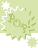Pop-ups: Choose the correct answer from a list of choices.# Logarithm Basics

Use the ^2 to mean exponent of 2, and ^3 to mean exponent of 3 and so on.
Use log5(6)= y to mean the log, base 5, of 6 = y . That is the 5 is the "small" subscript on the right side of the word "log". At the end copy your score and email them to me.This activity was created by a Quia Web subscriber. Learn more about QuiaCreate your own activities